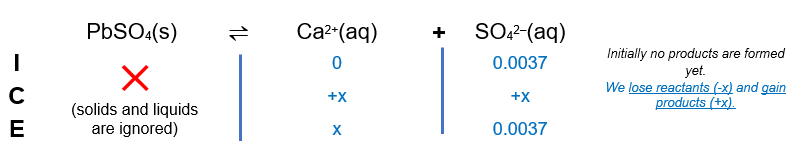# Problem: The Ksp of PbSO4 is 1.3 x 10-8. Calculate the solubility (in mol/L) of PbSO4 in a 0.0037 M solution of Na2SO4.

###### FREE Expert Solution

The Ksp of PbSO4 is 1.3 x 10-8. Calculate the solubility (in mol/L) of PbSO4 in a 0.0037 M solution of Na2SO4.

For this problem, we’re being asked to calculate the solubility (in g/L) of PbSO4(s) in 0.0037 M Na2SO4(aq). Since the compounds are ionic compounds, they form ions when dissociating in water. The dissociation of PbSO4 and Na2SO4 in water are as follows:

PbSO4(s)  Ca2+(aq) + SO42–(aq)

Na2SO4(s)  2 Na+(aq) + SO42–(aq)

Notice that there is a common ion present, SO42–. The common ion effect states that the solubility of a salt is lower in the presence of a common ion.

We can construct an ICE table for the dissociation of PbSO4. Remember that solids are ignored in the ICE table.85% (279 ratings)###### Problem Details

The Ksp of PbSO4 is 1.3 x 10-8. Calculate the solubility (in mol/L) of PbSO4 in a 0.0037 M solution of Na2SO4.